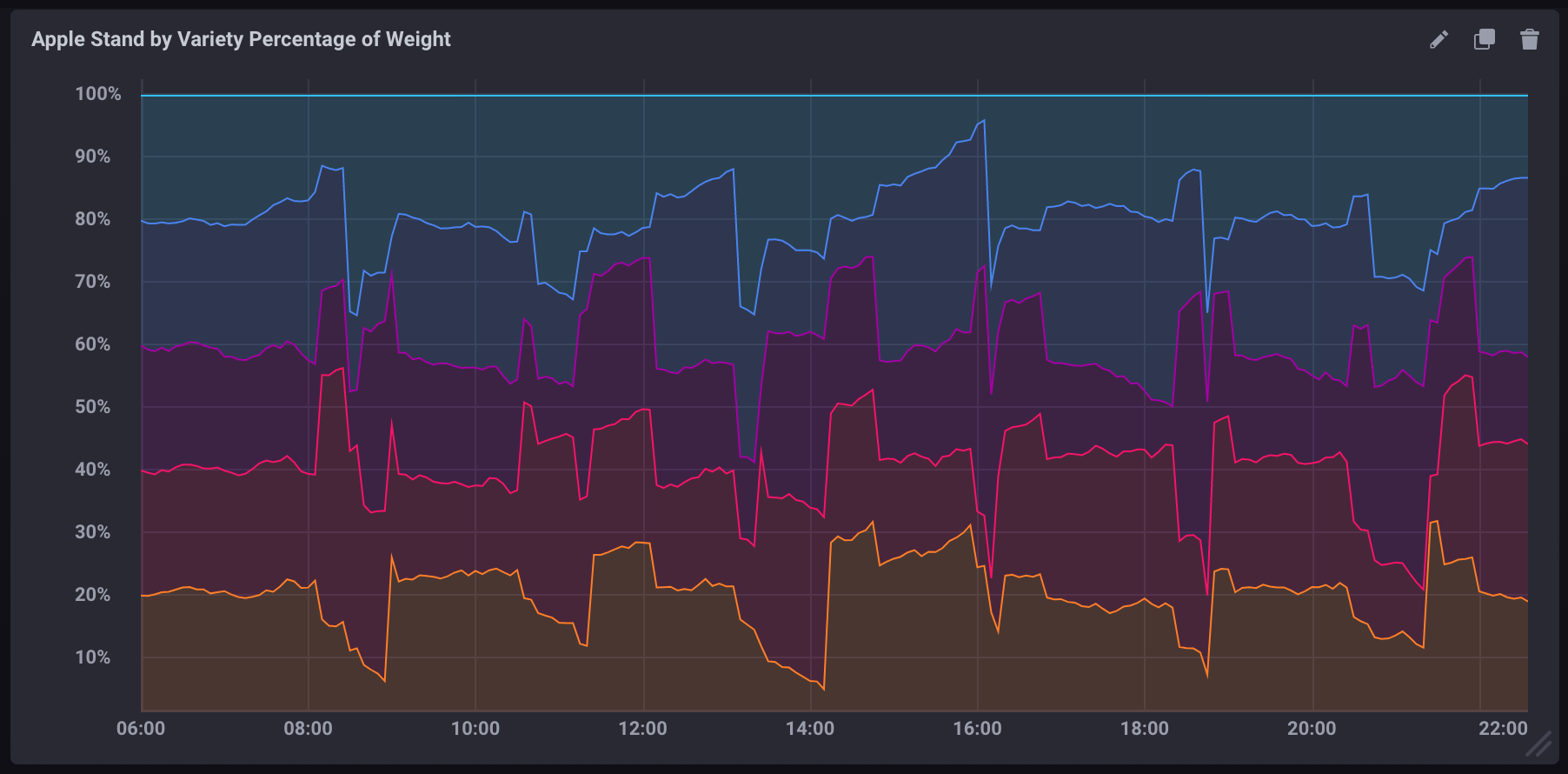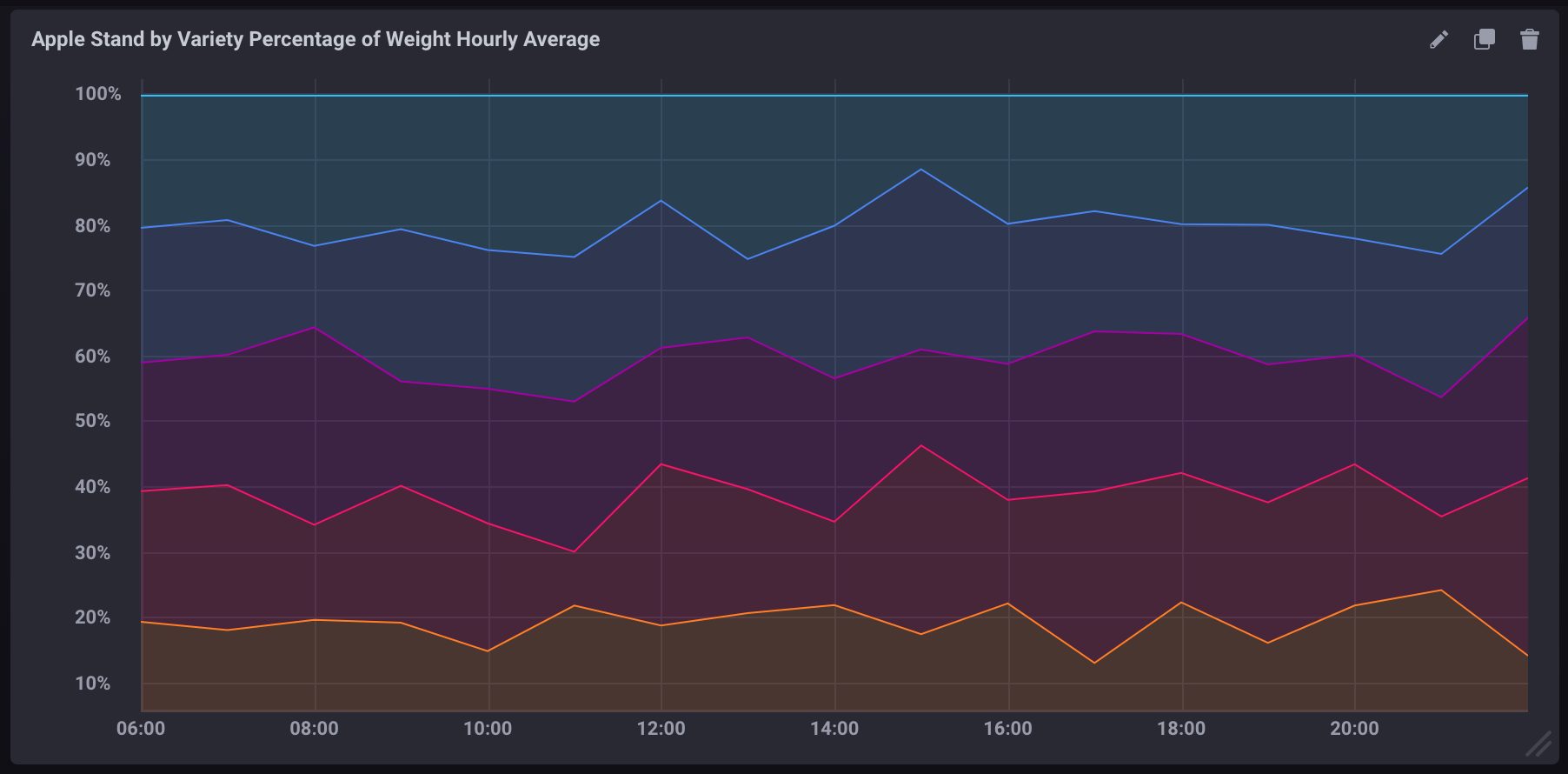Search

# 时间序列数据库 TSDB：计算查询中的百分比

InfluxQL允许您计算简单的数学方程式，这使得使用measurement中的两个field字段计算百分比非常简单。然而，有一些注意事项不能忽略。

# 查询中的基本计算

`SELECT`语句支持使用基本的数学运算符，例如，`+``-``/``*``()`等等。

``-- 两个field key相加``SELECT field_key1 + field_key2 AS "field_key_sum" FROM "measurement_name" WHERE time < now() - 15m``-- 两个field key相减``SELECT field_key1 - field_key2 AS "field_key_difference" FROM "measurement_name" WHERE time < now() - 15m``-- 分组计算并将它们连接起来``SELECT (field_key1 + field_key2) - (field_key3 + field_key4) AS "some_calculation" FROM "measurement_name" WHERE time < now() - 15m``

# 计算查询中的百分比

``SELECT (field_key1 / field_key2) * 100 AS "calculated_percentage" FROM "measurement_name" WHERE time < now() - 15m``

# 使用聚合函数计算百分比

``SELECT (sum(field_key1) / sum(field_key2)) * 100 AS "calculated_percentage" FROM "measurement_name" WHERE time < now() - 15m GROUP BY time(1m)``

# 示例

## 示例数据

1. 下载示例数据
2. 导入示例数据：

``influx -ssl -username <账号名称> -password <密码> -host <网络地址> -port 3242 -import -path=path/to/apple_stand.txt -database=apple_stand``

## 计算每个品种占总重量的百分比

``SELECT``    ("braeburn"/total_weight)*100,``    ("granny_smith"/total_weight)*100,``    ("golden_delicious"/total_weight)*100,``    ("fuji"/total_weight)*100,``    ("gala"/total_weight)*100``FROM "apple_stand"."autogen"."variety"``## 计算每个品种的聚合百分比

``SELECT``    (mean("braeburn")/mean(total_weight))*100,``    (mean("granny_smith")/mean(total_weight))*100,``    (mean("golden_delicious")/mean(total_weight))*100,``    (mean("fuji")/mean(total_weight))*100,``    (mean("gala")/mean(total_weight))*100``FROM "apple_stand"."autogen"."variety"``WHERE time >= '2018-06-18T12:00:00Z' AND time <= '2018-06-19T04:35:00Z'``GROUP BY time(1h)``

• 它使用聚合函数（`mean()`）来提取所有数据
• 它包含一个`GROUP BY time()`子句，将数据按一小时聚合
• 它包含一个明确的时间区间，如果没有它，聚合函数是非常耗费资源的InfluxDB® is a trademark registered by InfluxData, which is not affiliated with, and does not endorse, TSDB for InfluxDB®.